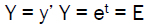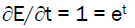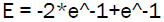All submissions of the EM system will be redirected to Online Manuscript Submission System. Authors are requested to submit articles directly to Online Manuscript Submission System of respective journal.

Paul TE Cusack*

Eng, Dule 1641, Sandy Point Rd, Saint John Nb, Canada

*Corresponding Author:
Paul TE Cusack
Eng, Dule 1641, Sandy Point Rd
Saint John Nb, Canada
E-mail: St-michael@hotmail.com

Received Date: 10/10/2016; Accepted Date: 22/10/2016; Published Date: 30/10/2016

Visit for more related articles at Research & Reviews: Journal of Statistics and Mathematical Sciences

Abstract

How did Nostradamus know the future? I examine how time trave is possible mathematically based on my previous work in Astro-Theology, Cusack’s Universe. Time-travel may be possible at a point when time stands still. It occurs when the dervative of the Energy parabola = 1, or when the rate of change of Energy is equal to the rate of change of time. This brief papers shows these calcualations and illustrations.

Keywords

Nostrasdamus, Time-travel, Astrotheology

Introduction

Time travel may be possible at only two points in History. The first precedes Christ; the second is when Nostradamus enters time on Dec 21, 1503 AD.

Consider that the Christian calendar is off by 4 to 6 years. We’ll assume 4 years for our calculations.

On the plot of Energy vs. time, the Golden Mean Energy parabola is illustrated in Figure 1 below. Assume that Jesus enetered time when the Energy parabola was mininum, or the derative was zero .Derivative 2t-1 = 0 t = 0.5

Scaling up by 1000, t = 500.

If we set the derivative = 1,t = 1, or scaling, t = 1000

Since the time between Jesus’ entry into time and Nostradamus’ entry is 1507, then,Consider Figure 2.Since t = π, and E = tConsider Figure 2.Derivative, -Pi = Ln Ln (Pi) = 1/PiThe derivative = 1, at t = 0.

This is the Ln function.Ln 1 = t

0 = t

Time stood still at ∂E/∂t = 1.

So time stood still when the derivative = 1

Consider Figure 3:So, let’s explore some more Math on time travel. For this, you’ll need to be acquainted with my theory on Astro-Theology, Cusack’s Universe.

The Clairnaut Equation for the Universe is:Taking the Integral of both sides, Integral G = ∂E/∂t = 1 for time travel

But, Newton tells us:

F = G M1M2/R

FR/(M1M2) = G

Integral G = Integral F R (M1M2)

If R, M1 and M2 are constant, the F = Ma turns into Momentum P = Mv

So PR/(M1M2) = 1

P = M1M2/R

VM2/ R

Letting R = s = 4/3

M = vs

M = (∂s/∂t)(s)

M/s = ∂s/∂t

R = M/s = 4.486/(4/3) = 3.3645 = 1/c

Therefore, R = 1/c where c is the speed of light.

Continuing with the Energy cone, (Figure 4),

Vol = 2 R2 *h/3

E = Vol = 2/ (3*(c2)

But E = Mc2

Ec2 = Mc2*c2

C4 = 1

C = 1

R = 1/c

R = 1

Now, Vol’ = Pi * R2 *h/3 & Vol’ = π (1)*h

Vol’ = Pπ*h

Let Vol = Vol’

Gh = πh

G = π = t

Refer to Figure 5.

t = G = π

E = 1/t

E = /G

G = Pi = E = 1/Pi = 31.8

Human Perception frequency when the frequency of the human brain is equal to the frequency of the Energy of the universe, time stands still.

And, Integral G = 1 = Integral (1/E) = Ln E = 1E = 1/t t = 368 From above t = R

Therefore, Space and time are equal allow for time travel. If we were to take a pill that allowed the human mind to operate at the same frequency as the universe, I think we would be able to travel through time. All time would converge to an instant. When G = 2/3 = 6.67, we have a Mass gap of 1.5 since E = 1/G = 1/t.

Conclusion

Nostradamus entered time when the derivative = 1. Time stood still for him on Dec 21, 1503 AD. Nostradamus was born at the exact right moment so that time stood still. Thus, he was able to travel through time, at least mathematically speaking. It may have been possible to travel through tame when This occurred before Jesus entered time on the other side of the Energy parabola. ∂E/∂t(-1). Time travel is possible mathematically speaking.